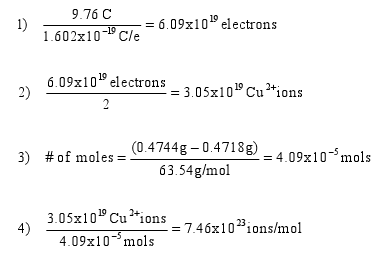Vernier Software & Technology
Innovative Uses

# Quick Determination of Avogadro's Number

This quick experiment is great for National Chemistry Week and celebrating Mole Day!

### OBJECTIVES

In this experiment, you will use an electrolytic cell to determine Avogadro’s number.

### MATERIALS

• Computer and Vernier computer interface or LabQuest
• Logger Pro 3 or LabQuest App
• Vernier Current Probe
• 3.0 volt DC power supply
• Four connecting wires with alligator clips
• Electrolyte solution (0.1 M H2SO4, salt optional)
• One 250 mL beaker
• Copper wire, ~ 0.5 g
• Balance (Sample data shown is taken with a 0.0001 g precision balance. If using a lower precision balance, run the experiment longer or at a higher voltage.)

### PROCEDURE

1. Weigh the copper wire pieces and record these masses.
2. Connect the alligator clips to the power supply and to the copper wire; using another alligator clip, connect the copper wire to the Current Probe making a complete circuit.
3. Place the copper wires into the beaker of electrolyte solution. Turn on the power supply to ~ 0.5 V.
4. Change the data collection length to 120 seconds and begin data collection.
5. When data collection is complete, dry and weigh the copper wire pieces and record these masses.

### PROCESSING THE DATA

1. We can easily determine the number of coulombs of electrical charge that have passed between the two electrodes using Logger Pro 3 or LabQuest, by taking the integral under the curve.
2. We know from Millikan’s oil-drop experiment that the charge on a single electron is 1.60218 x 10-19 coulombs. Therefore, the number of electrons that flowed between the two electrodes is: number of electrons = coulombs / (1.60218 x 10-19 coulomb/electron)
3. We have to correct for the fact that each copper atom releases two electrons when it is oxidized: number of Cu2+ ions = number of electrons / 2
4. Because we weighed the copper wires before and after the experiment, it is possible to determine the grams of copper (and therefore moles of copper) that were removed from the anode as Cu2+ ions: moles of Cu atoms = moles of Cu2+ ions = (initial anode mass – final anode mass) / (63.55 g/mol)
5. Lastly, divide the number of Cu2+ ions by the number of moles of Cu2+; this should result in a value similar to Avogadro’s number.

### QUESTIONS FOR DISCUSSION

1. In this cell, which is the anode and which is cathode? How can you tell?
2. What was your calculated value of Avogadro’s number and how close was it to the accepted value?
3. What caused the bubbles to form?
4. Why is an electrolyte solution necessary and not just tap water?

### SAMPLE DATA